# Bitwise operators

In all our examples below, we will check by using both binary and decimal numbers.
It is advisable to understand how to convert decimal to Binary and vice versa.

bin() : To convert integer to Binary
int() : To convert Binary to Integer ( base = 2 )
``````#printing decimal to binary
print(bin(120).replace('0b','')) # 1111000``````
``````# binary to decimal
print(int('1111000',2)) # 120``````

## Bitwise AND ( & )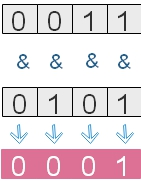Returns 1 when both operands are 1, otherwise returns 0.
``````print(3 & 4) # 0
print(bin(3).replace('0b','')) #  11
print(bin(4).replace('0b','')) # 100

print(12 & 15) # 12
print(bin(12).replace('0b','')) # 1100
print(bin(15).replace('0b','')) # 1111``````

## Bitwise OR ( | )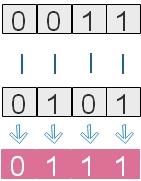Returns 0 if both operands 0, otherwise returns 1.
``````print(12 | 15) # 15
print(bin(12).replace('0b','')) # 1100
print(bin(15).replace('0b','')) # 1111

print(34 | 12) # 46
print(bin(34).replace('0b','')) # 100010
print(bin(12).replace('0b','')) #   1100
print(int('101110',2)) # 46``````

## Bitwise XOR ( ^ )Returns 1 of both operands are different, otherwise 0.
``````print(12 ^ 15) # 3
print(bin(12).replace('0b','')) # 1100
print(bin(15).replace('0b','')) # 1111

print(34 ^ 12) #  46
print(bin(34).replace('0b','')) # 100010
print(bin(12).replace('0b','')) #   1100
print(int('101110',2)) #  46``````

## 1's complement (~ )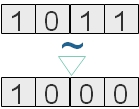Flips the bits until one 0 is reached (from right side )
This can be represented as
``~x = -x-1 ``
Examples
``````print(~15)  # -16
print(~4)   # -5
print(~-22) # 21
print(~99)  # -100``````

## Bitwise LEFT shift operator ( << )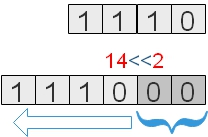This shifts left the binary number by given ( input ) places. The place vacated at left is filled by zeros.
``````print(14<<2)  # 56
print(bin(14).replace('0b','')) #1110``````

## Bitwise RIGHT shift operator ( >> )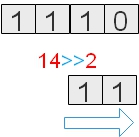This shifts right the binary number by given ( input ) places.
``````print(14>>2)  # 3
print(bin(14).replace('0b','')) #1110

print(90>>3)  # 11
print(bin(90).replace('0b','')) #1011010
print(int('1011',2)) #11``````
1. Examples of Bitwise operator
2. By using Left shift operator by 1 we can multiple the integer by 2
By using Right shift operator by 1 we can divide the integer by 2
``````print(14<<1)   # 28
print(14>>1)   # 7

print(100 <<1) # 200
print(100 >>1) # 50``````

Subscribe to our YouTube Channel here

## Subscribe

* indicates required
Subscribe to plus2netplus2net.com